# Fraction of a fraction

What is two-quarters of twelve?

x =  6

### Step-by-step explanation: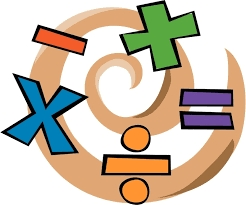Did you find an error or inaccuracy? Feel free to write us. Thank you!Tips to related online calculators
Need help to calculate sum, simplify or multiply fractions? Try our fraction calculator.

## Related math problems and questions:

• Ten fractionsWrite ten fractions between 1/3 and 2/3
• Minutes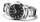Write as fraction in basic form which part of the week is 980 minutes.
• The machineThe machine was in operation for 9 tenths (in a fraction) of eight hours of working time. How long has he been running?
• Cake fractions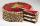Thomas ate 1/3 of cake, Bohouš of the rest of the cake ate 2/5. What fraction of cake left over for others?
• MilimetersHow many millimeters are 1/4 meters?
• Division by zero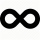Fraction 5 by 2. if 3 is added to numerator and 2 is subtracted from the denominator then the new fraction is:
• Equivalent fractions 2Write the equivalent multiplication expression. 2 1/6÷3/4
• Quotient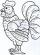Determine the quotient (q) and the remainder (r) from division numbers 100 and 8. Take the test of correctness.
• Two simple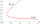Two simple fraction have the product of 3/10. When the smaller fraction is divided by the bigger fraction, the quotient is 5/6. What are the two fractions in simplest form?
• A quotientWhat is the quotient of 3/10 divided by 2/4 as a fraction?
• DivisionWhich number in division 16 give 12 and the rest 3?
• Fraction expressionWhich expression is equivalent to : minus 9 minus left parenthesis minus 4 start fraction 1 divided by 3 end fraction right parenthesis
• Women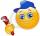In a company of 1050 employees are 2/3 women. How many women work in the company?
• Write 3Write a real-world problem involving the multiplication of a fraction and a whole number with a product that is between 8 and 10, then solve the problem.
• The average 3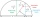The average of fraction is 7/10. One of the fractions is 2/3, the other fraction is?
• Two numbers and its productThe product of two numbers are 2/3. If on of them is 1/10, what is the other?
• Parenthesis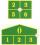Fill in the examples correctly brackets: A) 7.9+12:3-2 =23 B) 7.9+12:3-2=75